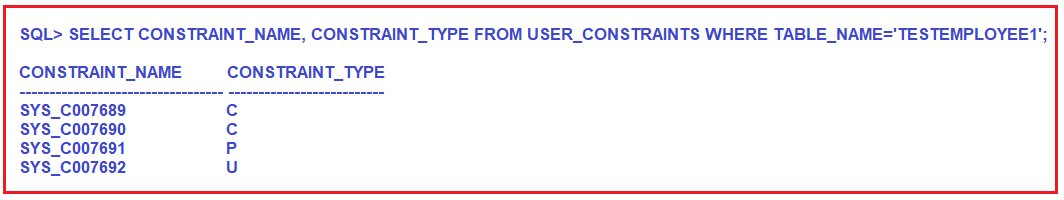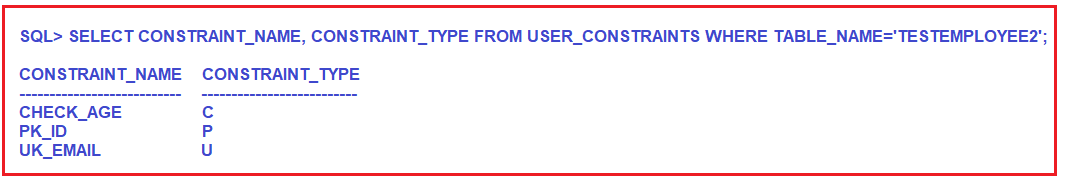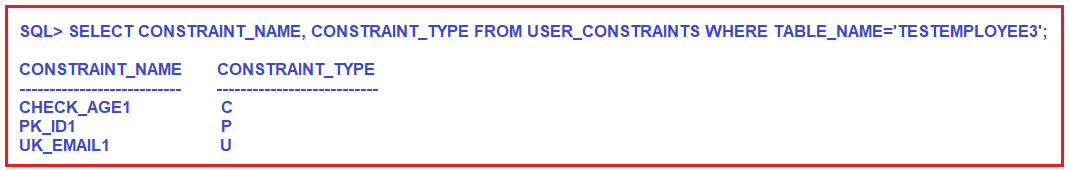# How to Create user defined Constraint name in Oracle

## How to Create Constraint with user-defined name in Oracle

In this article, I am going to discuss How to Create Constraints with user-defined names in Oracle with Examples. Please read our previous article where we discussed Data Dictionaries in Oracle with Examples. Before understanding How to Create user defined Constraint name in Oracle, let us first understand the Pre-Define Constraint Name in Oracle.

##### Pre-Define Constraint Name in Oracle:

Whenever we are applying constraint on a particular column then the Oracle database server(system) internally generates a unique id number (or) a unique constraint key name automatically for identifying a constraint. Let us understand this with an example.

##### Example to Understand Pre-Define Constraint Name in Oracle:

First, create the TestEmployee1 table by Executing the below CREATE TABLE statement. Here, you can see, we have applied three constraints i.e. Primary key on the Id column, the Unique key on the Email column, and the Check constraint on the Age column.

```CREATE TABLE TestEmployee1(
Id INT PRIMARY KEY,
Email VARCHAR2(20) UNIQUE,
Age INT NOT NULL CHECK (AGE >= 18)
);
```

Once you created the above TestEmployee1 table, lets us verify the constraint names by executing the below query. Here, I am providing the table name in the Upper case.

`SELECT CONSTRAINT_NAME, CONSTRAINT_TYPE FROM USER_CONSTRAINTS WHERE TABLE_NAME='TESTEMPLOYEE1';`

Once you execute the above query, you will get the following output. Here, C means CHECK constraints, P means Primary Key Constraints, and U means unique Key Constraints. Further, if you notice the names of the constraints are start with sys_ and then follow a unique alphanumeric string.Now, let us see, how to create a user-defined constraint name i.e. by giving a user-defined name to the constraints.

##### User Define Constraint Name in Oracle:

In place of a predefined constraint name, we can also create a user-defined constraint key name (or) constraint id for identifying a constraint. The following is the syntax to create a user-defined constraint in Oracle.

Syntax: <COLUMN NAME> <DT>[SIZE] CONSTRAINT <USER DEFINED CONSTRAINT NAME> <CONSTRAINT TYPE>

##### Example to understand user-defined Constraint name:

Let us create the TestEmployee2 table by Executing the below CREATE TABLE statement. Here, you can see, we have applied three constraints i.e. Primary key on the Id column, the Unique key on the Email column, and the Check Constraint on Age column. Further, if you notice we have provided the primary key constraint name as PK_ID, the unique key constraint name as UK_EMAIL, and the check constraint name as CHECK_AGE.

```CREATE TABLE TestEmployee2(
Id INT CONSTRAINT PK_ID PRIMARY KEY,
Email VARCHAR2(20) CONSTRAINT UK_EMAIL UNIQUE,
Age INT CONSTRAINT CHECK_AGE CHECK (AGE >= 18)
);
```

Once you created the above TestEmployee2 table, lets us verify the constraint names by executing the below query. Here, I am providing the table name in the Upper case.

`SELECT CONSTRAINT_NAME, CONSTRAINT_TYPE FROM USER_CONSTRAINTS WHERE TABLE_NAME='TESTEMPLOYEE2';`

Once you execute the above query, you will get the following output. Here, C means CHECK constraints, P means Primary Key Constraints, and U means unique Key Constraints. As you can see, here, it is displaying the user-defined constraint names.##### User-Defined Constraint Names at Table level:

The previous example that we have seen is defined as the user-defined constraint at the column level. Now, let us see how to define constraints at the table level by giving a user-defined constraint name. For better understanding, please have a look at the below CREATE TABLE statement. In the CREATE TABLE statement, we are defining the constraint at table level and while defining the constraint at table level, we are also providing a user-defined constraint name.

```CREATE TABLE TestEmployee3(
Id INT,
Email VARCHAR2(20),
Age INT,
CONSTRAINT PK_ID1 PRIMARY KEY (Id),
CONSTRAINT UK_EMAIL1 UNIQUE (Email),
CONSTRAINT CHECK_AGE1 CHECK (AGE >= 18)
);
```

Once you created the above TestEmployee3 table, lets us verify the constraint names by executing the below query.

`SELECT CONSTRAINT_NAME, CONSTRAINT_TYPE FROM USER_CONSTRAINTS WHERE TABLE_NAME='TESTEMPLOYEE3';`

Once you execute the above query, you will get the following output. Here, C means CHECK constraints, P means Primary Key Constraints, and U means unique Key Constraints. As you can see, here, it is displaying the user-defined constraint names which we provided while creating the table.In the next article, I am going to discuss How to add and drop constraints to an existing table in Oracle with Examples. Here, in this article, I try to explain How to Create user-defined Constraint names in Oracle with Examples and I hope you enjoy this How to Create user-defined Constraint names in Oracle article.